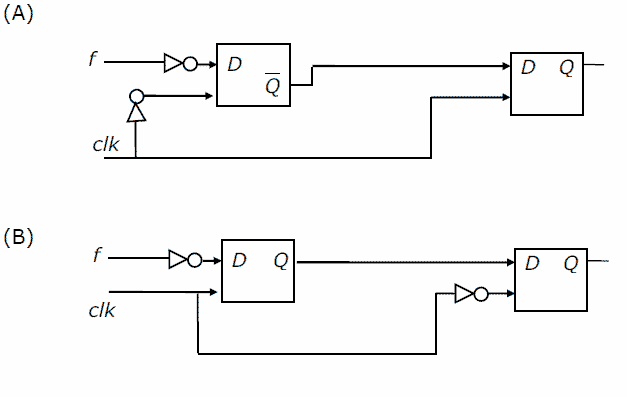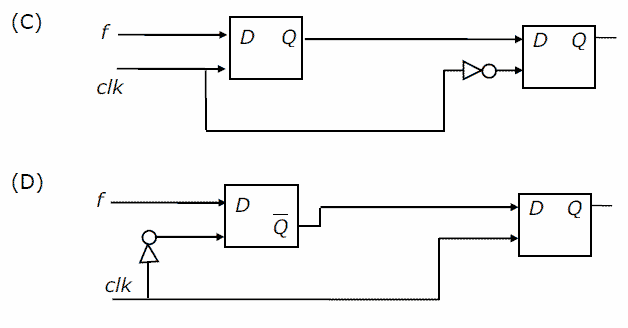# GATE | GATE-CS-2006 | Question 8

You are given a free running clock with a duty cycle of 50% and a digital waveform f which changes only at the negative edge of the clock. Which one of the following circuits (using clocked D flip-flops) will delay the phase of f by 180°?(A) A
(B) B
(C) C
(D) D

Explanation: We assume the D flip-flop to be negative edge triggered.

In option (A), during the negative edge of the clock, first flip-flop inverts complement of ‘f’. But, the output of first flip-flop has the same phase as ‘f’. Now, we give this output as input to the second flip-flop, which is enabled by ‘clk’.

Thus, we get a double inverted output having same phase as the input. So, A is not the correct option.

In option (B) and (D), the output is inverted ‘f’. But, we want ‘f’ as the output.
So, (B) and (D) can’t be the answer.

In option (C), the first flip-flop is activated by ‘clk’. So, the output of first flip-flop has the same phase as ‘f’. But, the second flip-flop is enabled by complement of ‘clk’. Since the clock ‘clk’ has a duty cycle of 50% , we get the output having phase delay of 180 degrees.

Therefore, (C) is the correct answer.

Please comment below if you find anything wrong in the above post.

Quiz of this Question

My Personal Notes arrow_drop_up
Article Tags :

Be the First to upvote.

Please write to us at contribute@geeksforgeeks.org to report any issue with the above content.